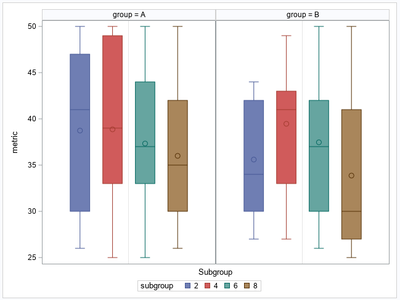## Can SGPANEL plot grouped VBOX and have a colaxis showing tic marks?

Consider this sample

``````data plotdata(keep=group id subgroup metric);
do group = 'A', 'B';
do subgroup = 2, 4, 6, 8;
do _n_ = 1 to 15;
id = (group='B') * 100 + _n_;
metric = rand('integer', 25, 50);
output;
end;
end;
end;
run;

proc sgpanel data=plotdata;
panelby group;
vbox metric / group = subgroup;
colaxis label='Subgroup' grid;
run;

ods html close;``````What is wanted is a plot showing the same boxes, but each group panel showing a colaxis with the subgroup values (2,4,6,8) as tic marks and the boxes above the marks.

I would turn off the legend with SGPANEL option NOAUTOLEGEND

1 ACCEPTED SOLUTION

Accepted SolutionsDanH_sas
SAS Super FREQ

## Re: Can SGPANEL plot grouped VBOX and have a colaxis showing tic marks?

After adding the CATEGORY option, you might also want to add the NOAUTOLEGEND option to the SGPANEL procedure statement. That way, the legend is suppressed. It does not add anything to the graph once you display the tick marks.

``````proc sgpanel data=plotdata noautolegend;
panelby group;
vbox metric / group = subgroup category = subgroup;
colaxis label='Subgroup' grid;
run;``````
2 REPLIES 2DanH_sas
SAS Super FREQ

## Re: Can SGPANEL plot grouped VBOX and have a colaxis showing tic marks?

``vbox metric / group = subgroup category = subgroup;``DanH_sas
SAS Super FREQ

## Re: Can SGPANEL plot grouped VBOX and have a colaxis showing tic marks?

After adding the CATEGORY option, you might also want to add the NOAUTOLEGEND option to the SGPANEL procedure statement. That way, the legend is suppressed. It does not add anything to the graph once you display the tick marks.

``````proc sgpanel data=plotdata noautolegend;
panelby group;
vbox metric / group = subgroup category = subgroup;
colaxis label='Subgroup' grid;
run;``````
Discussion stats
• 2 replies
• 258 views
• 1 like
• 2 in conversation